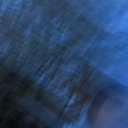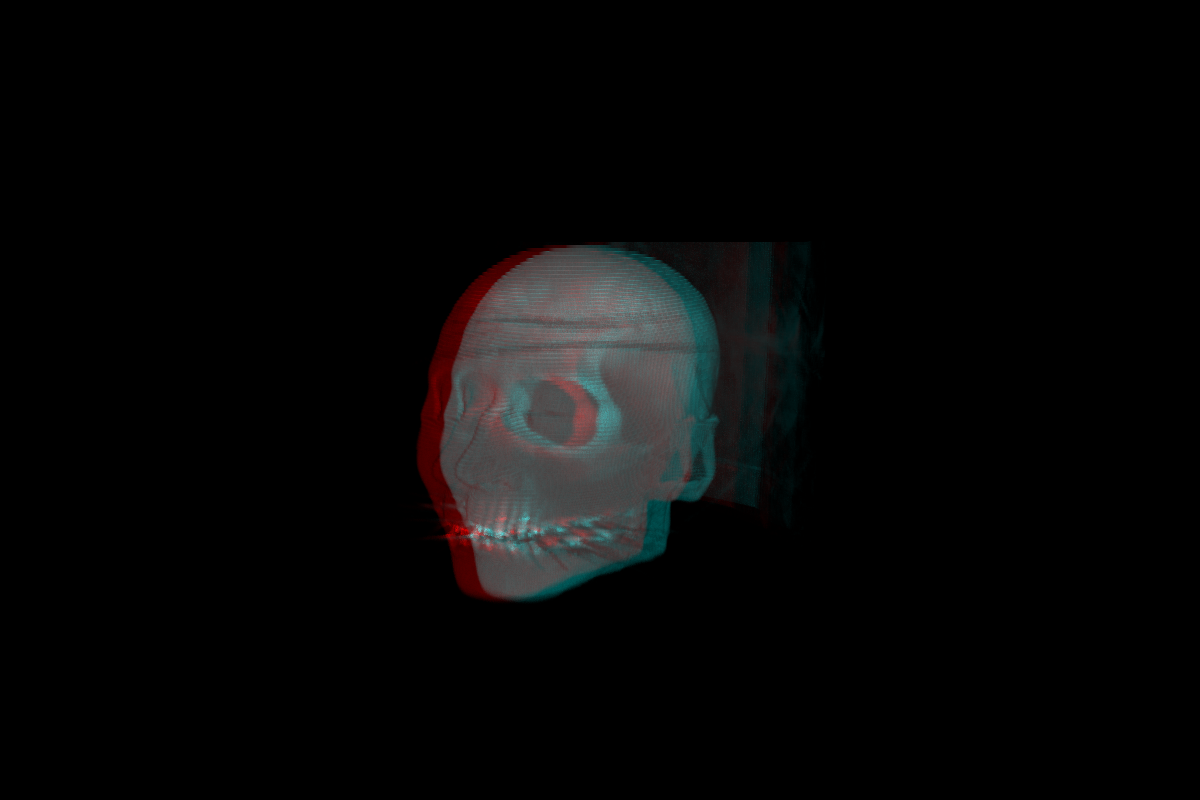•Jona

@ddw_music my mistake. i was not thinking in the fast prototyping way...
maybe was irritated by:

``````if type(window) ~= "userdata" then;
window = ofWindow();
end;
;
``````

do you still need that if you have an [of.window] object?

and:

"It turns out the real problem was a mistaken variable-name change in the fragment shader file. Once I corrected that, it went back to normal."

i feel you, that is one of my most common errors...•Jona

@ddw_music you always need a reference to the main ofelia object, something like:

``````local a = require("\$0-gameOfLife3d");
``````

see ofelia-object-help.pd -> variables.

and do not use M as a reference, because it is the same as ofelia...

•Jona

It is working.Made with Ofelia, shaders and the Open Frameworks addon ofxVolumetrics:

•Jona

@leonardo you can hide a bang with a canvas, perhaps you can hide it with [image] too?

•Jona

@ddw_music i think the 3d game of life shader works now. not that it is not supported, i just did not understand it.The only thing is, that ofFbo and ofTexture do not support 3d textures, but they need to be created with openGL (as far as I know, and what I did with help from ofxVolumetrics).
I only did not find a way to paint into the 3d texture yet, right know I need a .png image for every z dimension.
Here is a short example:

https://github.com/Jonathhhan/PureDataGameOfLife3d

•Jona

``````#version 120

uniform sampler3D Tex0;
uniform vec3 resolution;
uniform float lCell_0;
uniform float lCell_1;
uniform float lCell_2;
uniform float lCell_3;
uniform float lCell_4;
uniform float lCell_5;
uniform float lCell_6;
uniform float lCell_7;
uniform float lCell_8;
uniform float lCell_9;
uniform float lCell_10;
uniform float lCell_11;
uniform float lCell_12;
uniform float lCell_13;
uniform float lCell_14;
uniform float lCell_15;
uniform float lCell_16;
uniform float lCell_17;
uniform float lCell_18;
uniform float lCell_19;
uniform float lCell_20;
uniform float lCell_21;
uniform float lCell_22;
uniform float lCell_23;
uniform float lCell_24;
uniform float lCell_25;
uniform float lCell_26;
uniform float dCell_0;
uniform float dCell_1;
uniform float dCell_2;
uniform float dCell_3;
uniform float dCell_4;
uniform float dCell_5;
uniform float dCell_6;
uniform float dCell_7;
uniform float dCell_8;
uniform float dCell_9;
uniform float dCell_10;
uniform float dCell_11;
uniform float dCell_12;
uniform float dCell_13;
uniform float dCell_14;
uniform float dCell_15;
uniform float dCell_16;
uniform float dCell_17;
uniform float dCell_18;
uniform float dCell_19;
uniform float dCell_20;
uniform float dCell_21;
uniform float dCell_22;
uniform float dCell_23;
uniform float dCell_24;
uniform float dCell_25;
uniform float dCell_26;

vec2 rule = vec2(
vec2(lCell_0, dCell_0),
vec2(lCell_1, dCell_1),
vec2(lCell_2, dCell_2),
vec2(lCell_3, dCell_3),
vec2(lCell_4, dCell_4),
vec2(lCell_5, dCell_5),
vec2(lCell_6, dCell_6),
vec2(lCell_7, dCell_7),
vec2(lCell_8, dCell_8),
vec2(lCell_9, dCell_9),
vec2(lCell_10, dCell_10),
vec2(lCell_11, dCell_11),
vec2(lCell_12, dCell_12),
vec2(lCell_13, dCell_13),
vec2(lCell_14, dCell_14),
vec2(lCell_15, dCell_15),
vec2(lCell_16, dCell_16),
vec2(lCell_17, dCell_17),
vec2(lCell_18, dCell_18),
vec2(lCell_19, dCell_19),
vec2(lCell_20, dCell_20),
vec2(lCell_21, dCell_21),
vec2(lCell_22, dCell_22),
vec2(lCell_23, dCell_23),
vec2(lCell_24, dCell_24),
vec2(lCell_25, dCell_25),
vec2(lCell_26, dCell_26)
);

int get(int x, int y, int z) {
return int(texture3D(Tex0, (gl_FragCoord.xyz + vec3(x, y, z)) / resolution).r);
}

void main() {
int sum =
get(-1, -1, -1) +
get(-1,  0, -1) +
get(-1,  1, -1) +
get( 0, -1, -1) +
get( 0,  1, -1) +
get( 1, -1, -1) +
get( 1,  0, -1) +
get( 1,  1, -1) +
get(-1, -1, 0) +
get(-1,  0, 0) +
get(-1,  1, 0) +
get( 0, -1, 0) +
get( 0,  1, 0) +
get( 1, -1, 0) +
get( 1,  0, 0) +
get( 1,  1, 0) +
get(-1, -1, 1) +
get(-1,  0, 1) +
get(-1,  1, 1) +
get( 0, -1, 1) +
get( 0,  1, 1) +
get( 1, -1, 1) +
get( 1,  0, 1) +
get( 1,  1, 1);
vec2 r = rule[sum];
if (get(0, 0, 0) == 1) {
gl_FragColor = vec4(r.x, r.x, r.x, r.x);
}
else  {
gl_FragColor = vec4(r.y, r.y, r.y, r.y);
}
``````

But it does not work.Perhaps I did something wrong with the shader, but I think it does not work because ofFbo() can not store 3d textures.

•Jona

@alexandros for me compiling Ofelia with Ubuntu works without any problems and is quite fast.

posted in technical issues read more
•Jona

Second example, basically the same patch, but with ofxCameraAnaglyph for a 3d view...
https://github.com/Jonathhhan/volumetrics_anaglyph_example_ofelia
Here is a screenshot: